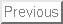Modality for Parabolic Group Actions

Gerhard Röhrle

Fakultät für Mathematik, Universität Bielefeld, Postfach 100131, 33501 Bielefeld, Germany.

roehrle@mathematik.uni-bielefeld.de

In this talk I shall discuss several aspects of the operation of parabolic subgroups of reductive groups.

Let G be a linear reductive algebraic group defined over an algebraically closed field k and let P be a parabolic subgroup of G. For instance if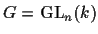, then P can be viewed as the stabilizer of a (partial) flag in kn. We consider the action of Pon its unipotent radical Pu via conjugation and on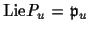, the Lie algebra of Pu, via the adjoint representation.

In 1974 R.W. Richardson proved that Padmits an open dense orbit on Pu, similarly for the adjoint action of P on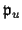. Thus there is a natural dichotomy: the instances when P acts onwith a finite number of orbits versus the cases when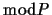is positive. Likewise for the action on Pu.

I shall give a complete description of all instances in classical goups when the number of P-orbits onis finite. Moreover, I shall present a complete combinatorial description of the relation of the P-orbit closures inin these finite instances for.

Time permitting we shall discuss the more general concept of the modality of the action of P on, denoted by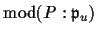. This is the maximal number of parameters upon which a family of P-orbits ondepends. Note that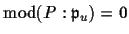precisely when P acts onwith a finite number of orbits.